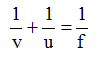# Physics

### Chapter : 4. Light Refraction

#### Numerical Method In Lens

Numerical Method In Lens
(A) LENS FORMULA
Definition : The equation relating the object distance (u), the image distance (v) and the focal length (f) of the lens is called the lens formula.
1. The lens is thin.
2. The lens has a small aperture.
3. The object lies close to principal axis.
4. The incident rays make small angles with the lens surface or the principal axis.
Lens Formula:(B) LINEAR MAGNIFICATION FOR LENS
LINEAR MAGNIFICATION
Definition : The ratio of the size of the image formed by refraction from the lens to the size of the object, is called linear magnification produced by the lens. It is represented by the symbol m.
If I be the size of the image and O be the size of the object, then(C) POWER OF LENS
Definition : It is the capacity or the ability of a lens to deviate (converge or diverge) the path of rays passing through it. A lens producing more converging or more diverging, is said to have more power